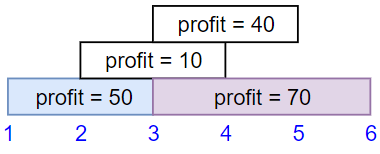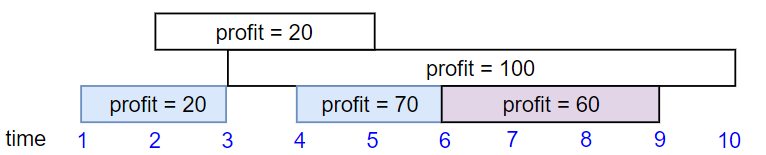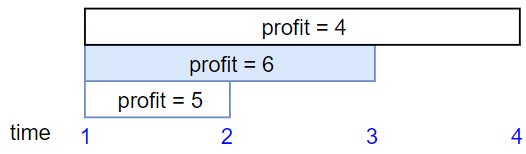## C# || How To Find The Maximum Profit In Job Scheduling Using C#The following is a module with functions which demonstrates how to find the maximum profit in job scheduling using C#.

1. Job Scheduling – Problem Statement

We have n jobs, where every job is scheduled to be done from startTime[i] to endTime[i], obtaining a profit of profit[i].

You’re given the startTime, endTime and profit arrays, return the maximum profit you can take such that there are no two jobs in the subset with overlapping time range.

If you choose a job that ends at time X you will be able to start another job that starts at time X.

Example 1:``` Input: startTime = [1,2,3,3], endTime = [3,4,5,6], profit = [50,10,40,70] Output: 120 Explanation: The subset chosen is the first and fourth job. Time range [1-3]+[3-6] , we get profit of 120 = 50 + 70. ```

Example 2:``` Input: startTime = [1,2,3,4,6], endTime = [3,5,10,6,9], profit = [20,20,100,70,60] Output: 150 Explanation: The subset chosen is the first, fourth and fifth job. Profit obtained 150 = 20 + 70 + 60. ```

Example 3:``` Input: startTime = [1,1,1], endTime = [2,3,4], profit = [5,6,4] Output: 6 ```

2. Job Scheduling – Solution

The following is a solution which demonstrates how to find the maximum profit in job scheduling.

``` 2. Job Scheduling - Solution C# // ============================================================================ // Author: Kenneth Perkins // Date: Jan 1, 2023 // Taken From: http://programmingnotes.org/ // File: Solution.cs // Description: Demonstrates how to find maximum profit in job scheduling // ============================================================================ public class Solution { public int JobScheduling(int[] startTime, int[] endTime, int[] profit) { if (startTime == null || startTime.Length == 0) { return 0; } var jobs = new List<Job>(); for (int index = 0; index < startTime.Length; ++index) { jobs.Add(new Job(startTime[index], endTime[index], profit[index])); } jobs.Sort((a, b) => a.Start - b.Start); return DFS(jobs, 0, new Dictionary<int, int>()); } private int DFS(List<Job> jobs, int currentJobIndex, Dictionary<int, int> maxProfits) { if (currentJobIndex >= jobs.Count) { return 0; } if (maxProfits.ContainsKey(currentJobIndex)) { return maxProfits[currentJobIndex]; } int next = BinarySearch(jobs, currentJobIndex); int inc = jobs[currentJobIndex].Profit + (next == -1 ? 0 : DFS(jobs, next, maxProfits)); int exc = DFS(jobs, currentJobIndex + 1, maxProfits); int maxProfit = Math.Max(inc, exc); maxProfits[currentJobIndex] = maxProfit; return maxProfit; } private int BinarySearch(List<Job> jobs, int currentJobIndex) { int lo = currentJobIndex; int high = jobs.Count - 1; int result = -1; while (lo <= high) { int mid = lo + (high - lo) / 2; if (jobs[currentJobIndex].End <= jobs[mid].Start) { result = mid; high = mid - 1; } else { lo = mid + 1; } } return result; } class Job { public int Start { get; set; } public int End { get; set; } public int Profit { get; set; } public Job(int startTime, int endTime, int profit) { Start = startTime; End = endTime; Profit = profit; } } }// http://programmingnotes.org/ 1234567891011121314151617181920212223242526272829303132333435363738394041424344454647484950515253545556575859606162636465666768697071 // ============================================================================//    Author: Kenneth Perkins//    Date:   Jan 1, 2023//    Taken From: http://programmingnotes.org///    File:  Solution.cs//    Description: Demonstrates how to find maximum profit in job scheduling// ============================================================================public class Solution {    public int JobScheduling(int[] startTime, int[] endTime, int[] profit) {        if (startTime == null || startTime.Length == 0) {            return 0;        }         var jobs = new List<Job>();        for (int index = 0; index < startTime.Length; ++index) {            jobs.Add(new Job(startTime[index], endTime[index], profit[index]));        }         jobs.Sort((a, b) => a.Start - b.Start);         return DFS(jobs, 0, new Dictionary<int, int>());    }     private int DFS(List<Job> jobs, int currentJobIndex, Dictionary<int, int> maxProfits) {        if (currentJobIndex >= jobs.Count) {            return 0;        }         if (maxProfits.ContainsKey(currentJobIndex)) {            return maxProfits[currentJobIndex];        }         int next = BinarySearch(jobs, currentJobIndex);        int inc = jobs[currentJobIndex].Profit + (next == -1 ? 0 : DFS(jobs, next, maxProfits));        int exc = DFS(jobs, currentJobIndex + 1, maxProfits);         int maxProfit = Math.Max(inc, exc);        maxProfits[currentJobIndex] =  maxProfit;        return maxProfit;    }     private int BinarySearch(List<Job> jobs, int currentJobIndex) {        int lo = currentJobIndex;        int high = jobs.Count - 1;        int result = -1;         while (lo <= high) {            int mid = lo + (high - lo) / 2;             if (jobs[currentJobIndex].End <= jobs[mid].Start) {                result = mid;                high = mid - 1;            } else {                lo = mid + 1;            }        }        return result;    }     class Job {        public int Start { get; set; }        public int End { get; set; }        public int Profit { get; set; }         public Job(int startTime, int endTime, int profit) {            Start = startTime;            End = endTime;            Profit = profit;        }    }}// http://programmingnotes.org/ ```

QUICK NOTES:
The highlighted lines are sections of interest to look out for.

The code is heavily commented, so no further insight is necessary. If you have any questions, feel free to leave a comment below.

Once compiled, you should get this as your output for the example cases:

``` 120 150 6 ```